## IF Function with Multiple Conditions

In this article, we will explore how IF function works in Excel. It is simple but very effective and useful. We will also explore how to add multiple conditions in an IF function. First we will explain how an IF function works, then we will go on to combine IF function with AND and OR functions. And at the end we will also look at nested IF functions.

## IF Function

The IF function in Excel allows us to:

• Have Excel examine a logical test. And if the test returns TRUE, we can have the function return a particular value which we get to specify. Alternatively if the test returns FALSE, we can have the function return another value which we also gets to specify.

The function is:

• =IF(logical test, [value if true], [value if false])

The square brackets around value if true and value if false do mean they are optional fields. The function will still work if nothing is entered for those two fields. In that case, TRUE will return 0 and FALSE will return FALSE.

Here’s an example below. We have a list of clients’ ages but the data is very hard to be presented because we could have data ranging from around 5 to 85. Using a simple IF function, we can group clients’ ages into “minor” or “adults”: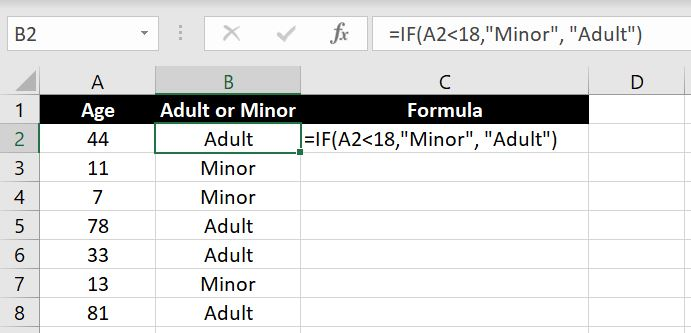Now we can use a COUNTIF function on column B to calculate the number of adults and minors in this list.

### Value If True and Value If False

With [value if true] and [value if false], we have set a string for each in the example above. However we can make it dynamic and have a formula there too. Here’s a simple example: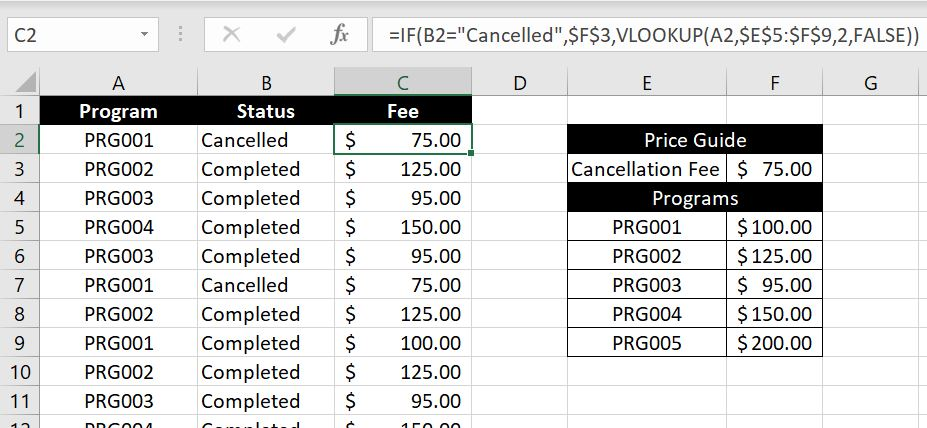The formula we have in C2 is:

• =IF(B2=”Cancelled”, \$F\$3, VLOOKUP(A2,\$E\$5:\$F\$9,2,FALSE))

Explanation: We first have Excel check the status in Column B. If status is “Cancelled”, the logical statement will be TRUE hence F3 – Cancellation Fee of \$75 will populate. If the status is not “Cancelled”, Excel will use VLOOKUP to search for the price in the Price Guide in E5:F9 for the relevant program in Column A.

### What Logical Tests Can We Include?

In the example above, we used “less than” as the logical test but there are so many more we could do in Excel. It would be impossible to list them all down but to list a few, we could:

• = : to compare two values
• < or >: “less than” or “greater than”
• <= or >=: “less than or equal to” or “greater than or equal to”
• ISBLANK: if a cell is blank
• ISNUMBER: if the value in a cell is a number
• ISTEXT: if the value in a cell is a text – note that if the value is a number or date, it will return FALSE

## IF & AND Functions

Essentially with IF functions, we enter a logical test and if it is TRUE, the [value if true] will return. Alternatively [value if false] will return. With the AND function, we can add multiple conditions into the logical test. And the logical test will only return TRUE if all conditions are met. As an example: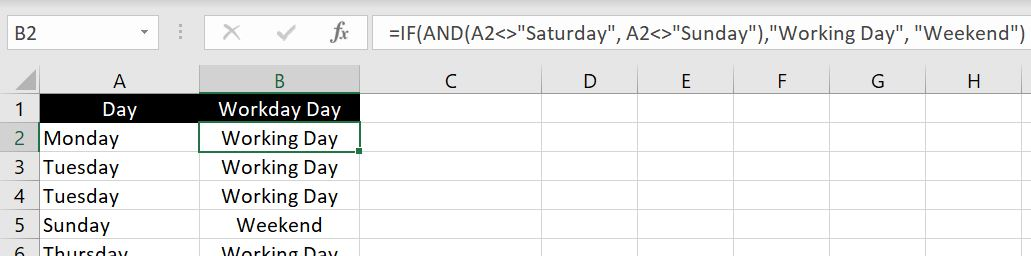• =IF(AND(A2<>”Saturday”, A2<>”Sunday”),”Working Day”, “Weekend”)

Explanation: in this case, two conditions must be met, the cell on the left must not be (<>) “Saturday” and must not be “Sunday”. If both conditions are met, “Working Day” will populate. Otherwise it will be “Weekend”. And of course you can add more than 2 conditions into the AND functions.

## IF & OR Functions

The OR function is very similar, except only one of the conditions needs to be met for the logical test to return TRUE. Let’s explore the same scenario above but this time we will use the OR function to reach the same outcome: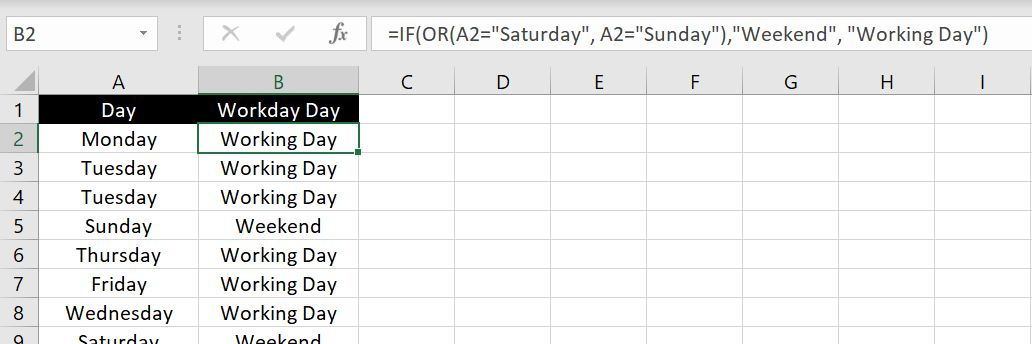• =IF(OR(A2=”Saturday”, A2=”Sunday”), “Weekend”, “Working Day”)

Explanation: in this case, only one of the logical tests in the OR function needs to be TRUE. That is, if the cell on the left is “Saturday” OR “Sunday”, the cell will be populated with “Weekend”. Otherwise it will be “Working Day”. And again, we can add more than 2 logical tests in OR functions.

## Nested IF Functions

So far we’ve only looked at IF functions with two possible outcomes. If TRUE then [value if true] will appear. If FALSE then [value if false] will appear. Things are not always so simple. What happens if you have more than 2 possible outcomes? Consider this example below:

If a staff is employed on a casual basis, there will be no bonus. There will also be no bonus if no KPIs are met. If one of the two KPIs are met and the staff is employed on a permanent basis, a Tier 2 bonus will be rewarded. And finally if both KPIs are met and the staff is employed on a permanent basis, a Tier 1 bonus will be rewarded: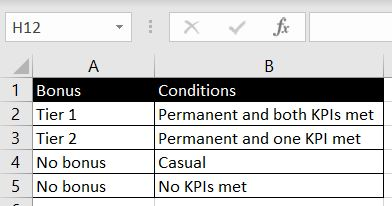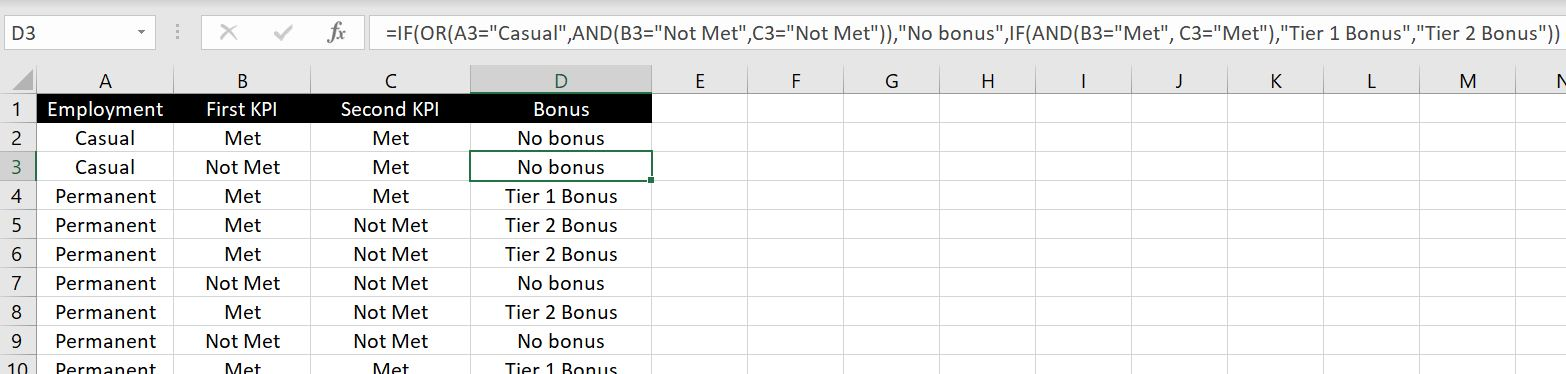Let’s break down this long formula:

• =IF(OR(A2=”Casual”, AND(B2=”Not Met”,C2=”Not Met”)),”No bonus”, IF(AND(B2=”Met”, C2=”Met”),”Tier 1 Bonus”, “Tier 2 Bonus”))
• =IF(OR(A2=”Casual”, AND(B2=”Not Met”,C2=”Not Met”)),”No bonus” : If staff is a casual OR if both KPIs are not yet, Excel will return “No bonus”. If this is not TRUE, then Excel will move onto the next IF function:
• IF(AND(B2=”Met”, C2=”Met”),”Tier 1 Bonus”, “Tier 2 Bonus”)): If the first logical test was not met then it would already mean that the employee is not a casual staff and the employee met one or both of the KPIs. In this next IF function, if both KPIs are met, Excel will return “Tier 1 Bonus”. Otherwise it would mean only one of the KPIs in which case “Tier 2 Bonus” will return.

## Nested IFs, AND and OR functions

As shown above with the AND and OR functions, we can sometimes reach the same outcome with either functions. We can also use nested IF functions to reach the same result. But imagine how many times we would have to nest the IF functions when we could perhaps group all conditions into an OR function. The question to ask is how many possible outcomes there are. If there are more than two, you will most likely need to use nested IF functions. But if there are only two possible outcomes, you should most likely be able to group all the conditions into AND and OR functions.